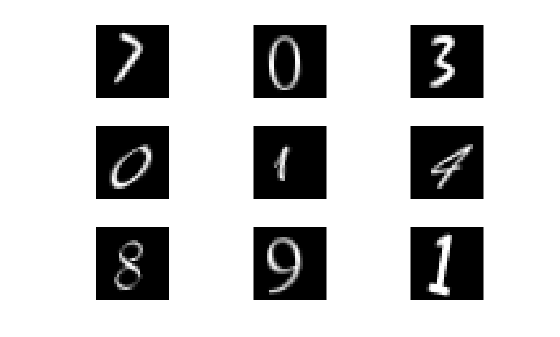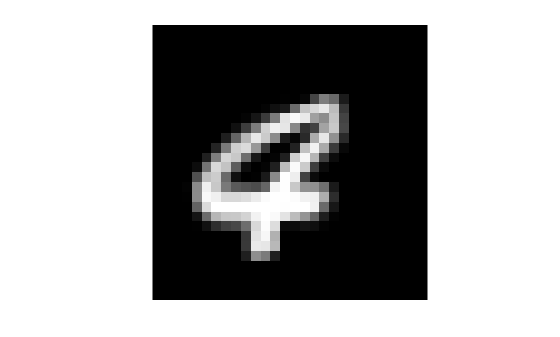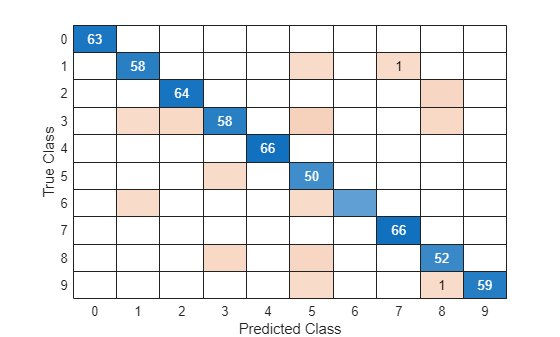Documentation

### This is machine translation

Mouseover text to see original. Click the button below to return to the English version of the page.

Note: This page has been translated by MathWorks. Click here to see
To view all translated materials including this page, select Country from the country navigator on the bottom of this page.

## Code Generation for Image Classification

This example shows how to generate C code from a MATLAB function that classifies images of digits using a trained classification model. This example demonstrates an alternative workflow to Digit Classification Using HOG Features (Computer Vision Toolbox). However, to support code generation in that example, you can follow the code generation steps in this example.

Automated image classification is an ubiquitous tool. For example, a trained classifier can be deployed to a drone to automatically identify anomalies on land in captured footage, or to a machine that scans handwritten zip codes on letters. In the latter example, after the machine finds the ZIP code and stores individual images of digits, the deployed classifier must guess which digits are in the images to reconstruct the ZIP code.

This example shows how to train and optimize a multiclass error-correcting output codes (ECOC) classification model to classify digits based on pixel intensities in raster images. The ECOC model contains binary support vector machine (SVM) learners. Then, this example shows how to generate C code that uses the trained model to classify new images. The data are synthetic images of warped digits of various fonts, which simulates handwritten digits.

### Set Up Your C Compiler

To generate C/C++ code, you must have access to a C/C++ compiler that is configured properly. MATLAB Coder locates and uses a supported, installed compiler. You can use `mex` `-setup` to view and change the default compiler. For more details, see Change Default Compiler (MATLAB).

### Assumptions and Limitations

To generate C code, MATLAB Coder:

• Requires a properly configured compiler.

• Requires supported functions to be in a MATLAB function that you define. For the basic workflow, see Introduction to Code Generation.

• Forbids objects as input arguments of the defined function.

Concerning the last limitation, consider that:

• Trained classification models are objects

• MATLAB Coder supports `predict` to classify observations using trained models, but does not support fitting the model

To work around the code generation limitations for classification, train the classification model using MATLAB, then pass the resulting model object to `saveCompactModel`. `saveCompactModel` reduces the memory footprint of the model (that is, makes it compact) if necessary, and then saves the trained model to disk as a structure array. Like the compact model, the structure array contains only the information used to classify new observations.

After saving the model to disk, load the model in the MATLAB function by using `loadCompactModel`. `loadCompactModel` loads the saved structure array, and then reconstructs the original compact model object. In the MATLAB function, to classify the observations, you can pass the model and predictor data set, which can be an input argument of the function, to `predict`.

### Code Generation for Classification Workflow

Before deploying an image classifier onto a device:

1. Obtain a sufficient amount of labeled images.

2. Decide which features to extract from the images.

3. Train and optimize a classification model. This step includes choosing an appropriate algorithm and tuning hyperparameters, that is, model parameters not fit during training.

4. Save the model to disk by using `saveCompactModel`.

5. Define a function for classifying new images. The function must load the model by using `loadCompactModel`, and can return labels, such as classification scores.

6. Set up your C compiler.

7. Decide the environment in which to execute the generated code.

8. Generate C code for the function.

### Load Data

Load the `digitimages` data set.

`load digitimages`

`images` is a 28-by-28-by-3000 array of `uint16` integers. Each page is a raster image of a digit. Each element is a pixel intensity. Corresponding labels are in the 3000-by-1 numeric vector `Y`. For more details, enter `Description` at the command line.

Store the number of observations and number of predictor variables. Create a data partition that specifies to hold out 20% of the data. Extract training and test set indices from the data partition.

```rng(1) % For reproducibility n = size(images,3); p = numel(images(:,:,1)); cvp = cvpartition(n,'Holdout',0.20); idxTrn = training(cvp); idxTest = test(cvp);```

Display nine random images from the data.

```figure for j = 1:9 subplot(3,3,j) selectImage = datasample(images,1,3); imshow(selectImage,[]) end```### Rescale Data

Because raw pixel intensities vary widely, you should normalize their values before training a classification model. Rescale the pixel intensities so that they range in the interval [0,1]. That is, suppose ${p}_{ij}$ is pixel intensity $j$ within image $i$. For image $i$, rescale all of its pixel intensities using this formula:

`${\underset{}{\overset{ˆ}{p}}}_{ij}=\frac{{p}_{ij}-\underset{j}{\mathrm{min}}\left({p}_{ij}\right)}{\underset{j}{\mathrm{max}}\left({p}_{ij}\right)-\underset{j}{\mathrm{min}}\left({p}_{ij}\right)}.$`

```X = double(images); for i = 1:n minX = min(min(X(:,:,i))); maxX = max(max(X(:,:,i))); X(:,:,i) = (X(:,:,i) - minX)/(maxX - minX); end```

Alternatively, if you have an Image Processing Toolbox™ license, then you can efficiently rescale pixel intensities of images to [0,1] by using `mat2gray`. For more details, see `mat2gray`.

### Reshape Data

For code generation, the predictor data for training must be in a table of numeric variables or a numeric matrix.

Reshape the data to a matrix such that predictor variables (pixel intensities) correspond to columns, and images (observations) to rows. Because `reshape` takes elements column-wise, you must transpose its result.

`X = reshape(X,[p,n])';`

To ensure that preprocessing the data maintains the image, plot the first observation in `X`.

```figure imshow(reshape(X(1,:),sqrt(p)*[1 1]),[],'InitialMagnification','fit')```### Extract Features

Computer Vision System Toolbox™ offers several feature-extraction techniques for images. One such technique is the extraction of histogram of oriented gradient (HOG) features. To learn how to train an ECOC model using HOG features, see Digit Classification Using HOG Features (Computer Vision Toolbox). For details on other supported techniques, see Local Feature Detection and Extraction (Computer Vision Toolbox). This example uses the rescaled pixel intensities as predictor variables.

### Train and Optimize Classification Model

Linear SVM models are often applied to image data sets for classification. However, SVM are binary classifiers, and there are 10 possible classes in the data set.

You can create a multiclass model of multiple binary SVM learners using `fitcecoc`. `fitcecoc` combines multiple binary learners using a coding design. By default, `fitcecoc` applies the one-versus-one design, which specifies training binary learners based on observations from all combinations of pairs of classes. For example, in a problem with 10 classes, `fitcecoc` must train 45 binary SVM models.

In general, when you train a classification model, you should tune the hyperparameters until you achieve a satisfactory generalization error. That is, you should cross-validate models for particular sets of hyperparameters, and then compare the out-of-fold misclassification rates.

You can choose your own sets of hyperparameter values, or you can specify to implement Bayesian optimization. (For general details on Bayesian optimization, see Bayesian Optimization Workflow.) This example performs cross-validation over a chosen grid of values.

To cross-validate an ECOC model of SVM binary learners based on the training observations, use 5-fold cross-validation. Although the predictor values have the same range, to avoid numerical difficulties during training, standardize the predictors. Also, optimize the ECOC coding design and the SVM box constraint. Use all combinations of these values:

• For the ECOC coding design, use one-versus-one and one-versus-all.

• For the SVM box constraint, use three logarithmically-spaced values from 0.1 to 100 each.

For all models, store the 5-fold cross-validated misclassification rates.

```coding = {'onevsone' 'onevsall'}; boxconstraint = logspace(-1,2,3); cvLoss = nan(numel(coding),numel(boxconstraint)); % For preallocation for i = 1:numel(coding) for j = 1:numel(boxconstraint) t = templateSVM('BoxConstraint',boxconstraint(j),'Standardize',true); CVMdl = fitcecoc(X(idxTrn,:),Y(idxTrn),'Learners',t,'KFold',5,... 'Coding',coding{i}); cvLoss(i,j) = kfoldLoss(CVMdl); fprintf('cvLoss = %f for model using %s coding and box constraint=%f\n',... cvLoss(i,j),coding{i},boxconstraint(j)) end end```
```cvLoss = 0.052083 for model using onevsone coding and box constraint=0.100000 cvLoss = 0.055000 for model using onevsone coding and box constraint=3.162278 cvLoss = 0.050000 for model using onevsone coding and box constraint=100.000000 cvLoss = 0.116667 for model using onevsall coding and box constraint=0.100000 cvLoss = 0.123750 for model using onevsall coding and box constraint=3.162278 cvLoss = 0.125000 for model using onevsall coding and box constraint=100.000000 ```

Determine the hyperparameter indices that yield the minimal misclassification rate. Train an ECOC model using the training data. Standardize the training data and supply the observed, optimal hyperparameter combination.

`minCVLoss = min(cvLoss(:))`
```minCVLoss = 0.0500 ```
```linIdx = find(cvLoss == minCVLoss); [bestI,bestJ] = ind2sub(size(cvLoss),linIdx); bestCoding = coding{bestI}```
```bestCoding = 'onevsone' ```
`bestBoxConstraint = boxconstraint(bestJ)`
```bestBoxConstraint = 100 ```
```t = templateSVM('BoxConstraint',bestBoxConstraint,'Standardize',true); Mdl = fitcecoc(X(idxTrn,:),Y(idxTrn),'Learners',t,'Coding',bestCoding);```

Construct a confusion matrix for the test set images.

```testImages = X(idxTest,:); testLabels = predict(Mdl,testImages); confusionMatrix = confusionchart(Y(idxTest),testLabels);```Diagonal and off-diagonal elements correspond to correctly and incorrectly classified observations, respectively. `Mdl` seems to correctly classify most images.

If you are satisfied with the performance of `Mdl`, then you can proceed to generate code for prediction. Otherwise, you can continue adjusting hyperparameters. For example, you can try training the SVM learners using different kernel functions.

### Save Classification Model to Disk

`Mdl` is a predictive classification model, but you must prepare it for code generation. Save `Mdl` to your present working directory using `saveCompactModel`.

`saveCompactModel(Mdl,'DigitImagesECOC')`

`saveCompactModel` compacts `Mdl`, converts it to a structure array, and saves it in the MAT-file `DigitImagesECOC.mat`.

### Define Prediction Function for Code Generation

Define an entry-point function named `predictDigitECOC.m`. that does the following:

• Include the code generation directive `%#codegen` somewhere in the function.

• Accept image data commensurate with `X`.

• Load `DigitImagesECOC.mat` using `loadCompactModel`.

• Return predicted labels.

`type predictDigitECOC.m % Display contents of predictDigitECOC.m file`
```function label = predictDigitECOC(X) %#codegen %PREDICTDIGITECOC Classify digit in image using ECOC Model % PREDICTDIGITECOC classifies the 28-by-28 images in the rows of X using % the compact ECOC model in the file DigitImagesECOC.mat, and then % returns class labels in label. CompactMdl = loadCompactModel('DigitImagesECOC.mat'); label = predict(CompactMdl,X); end ```

Note: If you click the button located in the upper-right section of this page and open this example in MATLAB®, then MATLAB® opens the example folder. This folder includes the entry-point function file.

Verify that the prediction function returns the same test set labels as `predict`.

```pfLabels = predictDigitECOC(testImages); verifyPF = isequal(pfLabels,testLabels)```
```verifyPF = logical 1 ```

`isequal` returns logical 1 (`true`), which means all the inputs are equal. The `predictDigitECOC` yields the expected results.

### Decide Which Environment to Execute Generated Code

Generated code can run:

• Inside the MATLAB environment as a C-MEX file

• Outside the MATLAB environment as a standalone executable

• Outside the MATLAB environment as a shared utility linked to another standalone executable

This example generates a MEX file to be run in the MATLAB environment. Generating such a MEX file allows you to test the generated code using MATLAB tools before deploying the function outside the MATLAB environment. In the MEX function, you can include code for verification, but not for code generation, by declaring the commands as extrinsic using `coder.extrinsic`. Extrinsic commands can include functions that do not have code generation support. All extrinsic commands in the MEX function run in MATLAB, but `codegen` does not generate code for them.

If you plan to deploy the code outside the MATLAB environment, then you must generate a standalone executable. One way to specify your compiler choice is by using the `-config` option of `codegen`. For example, to generate a static C executable, specify `-config:exe` when you call codegen. For more details on setting code generation options, see the `-config` option of `codegen`.

### Compile MATLAB Function to MEX File

Compile `predictDigitECOC.m` to a MEX file using `codegen`. Specify these options:

• `-report` — Generates a compilation report that identifies the original MATLAB code and the associated files that `codegen` creates during code generation.

• `-args` — MATLAB Coder requires that you specify the properties of all the function input arguments. One way to do this is to provide `codegen` with an example of input values. Consequently, MATLAB Coder infers the properties from the example values. Specify the test set images commensurate with `X`.

`codegen predictDigitECOC -report -args {testImages}`
```Code generation successful: View report ```

`codegen` successfully generated the code for the prediction function. You can view the report by clicking the link at the command line. If code generation is unsuccessful, then the report can help you debug.

`codegen` creates the directory `pwd/codegen/mex/predictDigitECOC`, where `pwd` is your present working directory. In the child directory, `codegen` generates, among other things, the MEX-file `predictDigitECOC_mex.mexw64`.

Verify that the MEX file returns the same labels as `predict`.

```mexLabels = predictDigitECOC_mex(testImages); verifyMEX = isequal(mexLabels,testLabels)```
```verifyMEX = logical 1 ```

`isequal` returns logical 1 (`true`), meaning that the MEX-file yields the expected results.

Download ebook